### SOLUTIONS TO U-SUBSTITUTION

SOLUTION 8 : Integrate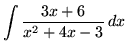. Let

u = x2+4x-3

so that

du = (2x+4) dx = 2 (x+2) dx ,

or

(1/2) du = (x+2) dx .

Substitute into the original problem, replacing all forms of x, getting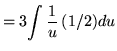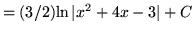.

SOLUTION 9 : Integrate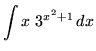. Let

u = x2+1

so that

du = 2x dx ,

or

(1/2) du = x dx .

Substitute into the original problem, replacing all forms of x, getting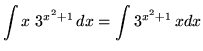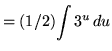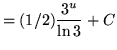(Recall that.)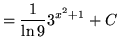.

SOLUTION 10 : Integrate. Letso that.

Substitute into the original problem, replacing all forms of x, getting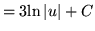.

SOLUTION 11 : Integrate. Letso that (Don't forget to use the chain rule.)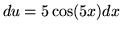,

or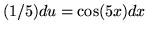.

Substitute into the original problem, replacing all forms of x, getting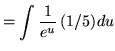(Do not make the following VERY COMMON MISTAKE :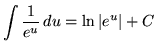. Why is this INCORRECT ?)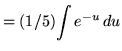.

Now make another substitution. Let

w = -u

so that

dw = (-1) du ,

or

(-1) dw = du .

Substitute into the problem, replacing all forms of u, getting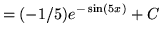.

SOLUTION 12 : Integrate. Let

u = x2

so that

du = 2x dx ,

or

(1/2) du = x dx .

In addition, the range of x-values is,

so that the range of u-values is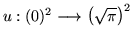,

or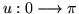.

Substitute into the original problem, replacing all forms of x and the x-values, getting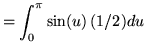= (-1/2)( (-1) - (1) )

= (-1/2)( -2)

= 1 .

SOLUTION 13 : Integrate. Let

u = x-1

so that

du = (1) dx = dx .

In addition, we can "back substitute" with

x = u+1 .

Substitute into the original problem, replacing all forms of x, getting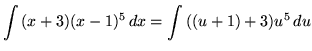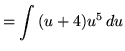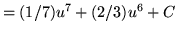.